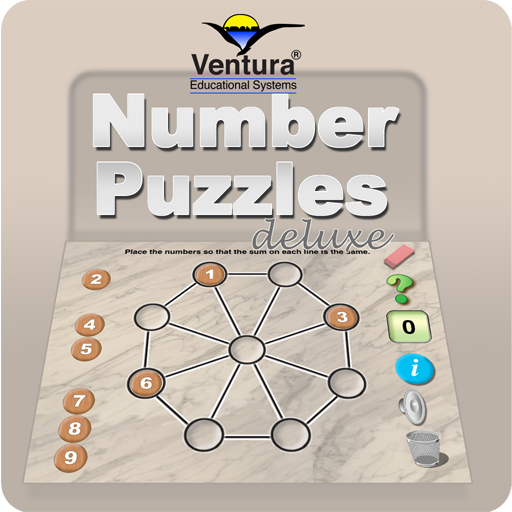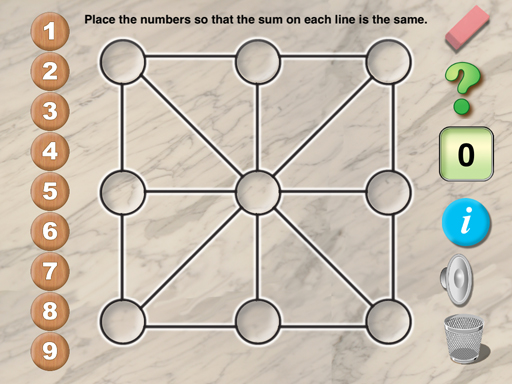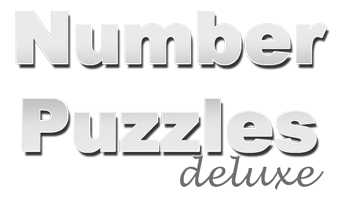A New and Exciting Puzzle with Fun Features!Interactive Excitement in a Challenging Puzzle
 Number Puzzles Deluxe is a collection of virtual manipulative problems that requires students to arrange numbers in certain positions so that the sums in different directions are the same. There are 5 puzzles in this collection. The puzzles are appropriate for elementary age students but fun for anyone who enjoys math puzzles. The object is to place numbers from 1 to 9 in a pattern so that the sum along each line of the design is the same. For example, in one puzzle students place numbers along the sides of an equilateral triangle so that the sum is equal to one of three different numbers. Each puzzle requires a different logical strategy. Because the app is design to simulate a table top with manipulative, students are encouraged to experiment. There is educational value in just letting students play with the puzzles and do the mental arithmetic involved in finding the sum, thus, reinforcing addition skills. Teachers will find it beneficial to have students verbalize the mathematical thinking and logical reasoning that they are using to solve the puzzle.Special features make using Number Puzzles Deluxe fun: 1. Slide numbers into place to solve puzzle. 2. Random puzzles automatically generated. 3. Sound effects. 4. Available for iPad iOS 7 and 8.\$0.99